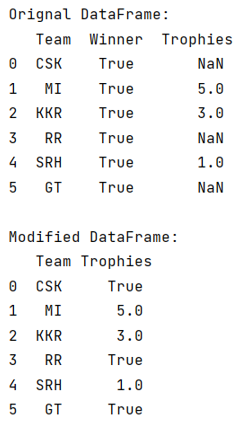# Python Pandas: Replace NaN in one column with value from corresponding row of second column

Given a Pandas DataFrame, we have to replace NaN in one column with value from corresponding row of second column.
Submitted by Pranit Sharma, on June 22, 2022

Pandas is a special tool that allows us to perform complex manipulations of data effectively and efficiently. Inside pandas, we mostly deal with a dataset in the form of DataFrame. DataFrames are 2-dimensional data structures in pandas. DataFrames consist of rows, columns, and the data.

While creating a DataFrame or importing a CSV file, there could be some NaN values in the cells. NaN values mean "Not a Number" which generally means that there are some missing values in the cell. To deal with this type of data, you can either remove the particular row (if the number of missing values is low) or you can handle these values.

To replace NaN values in one column with values from the corresponding row of the second column, we will first fill and replace all the NaN values with the corresponding values of the second column, and after that, we will delete the other column to prevent data redundancy.

To work with pandas, we need to import pandas package first, below is the syntax:

```import pandas as pd
```

Let us understand with the help of an example,

## Python Code to replace NaN in one column with value from corresponding row of second column

```# Importing pandas package
import pandas as pd

# Importing numpy package
import numpy as np

# Create a DataFrame
df = pd.DataFrame({
'Team':['CSK','MI','KKR','RR','SRH','GT'],
'Winner':[True,True,True,True,True,True],
'Trophies':[np.NaN,5,3,np.NaN,1,np.NaN]
})

# Display original DataFrame
print("Orignal DataFrame:\n",df,"\n")

# Replace values of Trophies with winner values

# Delete Winner column
del df['Winner']

# Display result
print("Modified DataFrame:\n",df)
```

Output: